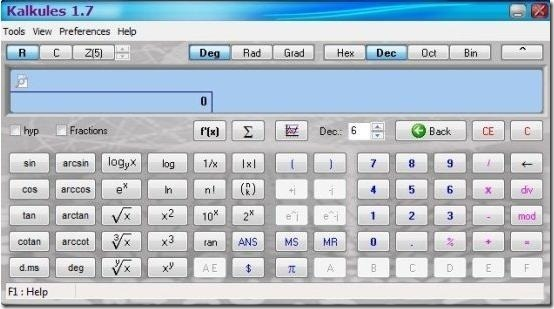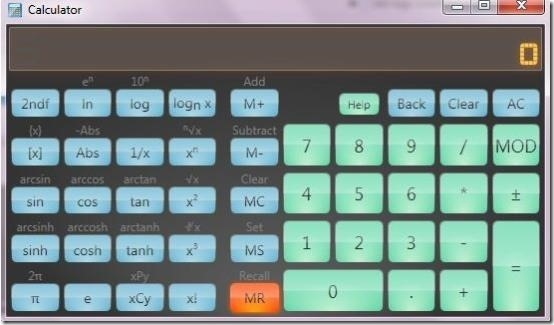Editor Ratings:
User Ratings:
[Total: 0 Average: 0]

Here is a list of 5 free scientific calculator software for PC that can be used for complex and advanced mathematical calculations.

Students at high school or university have to do difficult calculations and sometimes it becomes messy and irritating while calculating the expressions or equations manually. Keeping this in mind, I’ve reviewed some free scientific calculator software which will definitely ease your calculations. These calculators deal with elementary maths, trigonometry, exponential, arithmetic, hyperbolic functions, etc. These free scientific calculators have handy interface and provide multiple constants, functions, variables, and more.

Below I’ve reviewed Tinger Calc, RedCrab, Kalkules scientific calculator, Tibi’s Mathematics Suite, and SpeedCrunch.

## Tinger Calc:Tinger Calc is a free scientific calculator software for PC that lets you perform complex calculations along with some difficult mathematical operations. It can easily deal with elementary maths, trigonometric, and exponential functions, and it allows you to use mathematical constants like Pi and e. This free scientific calculator software lets you perform mathematical calculations with ease and without any difficulty.

## RedCrab:RedCrab is a free scientific calculator software that lets you perform complex and difficult mathematical calculations. It provides you full screen editor which supports corrections, editing, saving as well as printing. This free scientific calculator software allows you to enter all types of complex equations and formulas, and lets you use the result in subsequent calculations. It supports multiple types of mathematical calculations that include pi, trigonometric functions, power, f(x), Mod, Square root, Div, Hex, log, and more. In addition, this scientific calculator software allows you to import images and text into the formula editing section, to perform corrections if any, or for better explanation.

## Kalkules scientific calculator:Kalkules scientific calculator is a free scientific calculator software that offers you variety of tools so as to quickly do your calculations. This free scientific calculator software lets you perform complex and difficult calculations, as it has the ability to evaluate complex equations/expressions with variables, parentheses, functions, and constants. This freeware lets you calculate with complex numbers, real numbers, modulo numbers, fractions, and polynomials. Not only that, it also offers you variety of goniometric, arithmetic and hyperbolic functions. It offers you unit conversion feature, expression libraries, evaluation history, and more.

## Tibi’s Mathematics Suite:Tibi’s Mathematics Suite is a free maths suite that offers you free scientific calculator, graphing calculator, matrix calculator, and numeric factorizator. It offers you a free scientific calculator, which you can use to perform various advanced mathematical calculations. Matrix calculator lets you calculate matrix problems with ease, allowing you to consider two matrices at a time. Graphing calculator allows lets you to type in expressions and allows you to plot, evaluate, or integrate, accordingly. With the help of numeric factorization, you can perform factorization and compute the result. This free maths suite sits in your system tray quietly and lets you select the desired option simply via right click on the icon.

## SpeedCrunch:SpeedCrunch is a free scientific calculator software that lets you perform various complex and advanced mathematical calculations. It provides you preset functions, variables, and constants, which can be easily used while calculations. This free scientific calculator software calculates while typing and provides you instant quick results. It provides results that are up to 50 decimal points. It offers you syntax highlighting feature which lets you minimize errors as you type expressions, equations, and other complex mathematical calculations. It records every step in the history so that you can easily recall the steps and reuse later. It lets you save mathematical calculations in SCH format and on the other hand it allows you to export your work in TXT or HTML file format, which can be later on used with this free scientific calculator utility.

Students struggling with complex and difficult mathematical calculations can try out these free scientific calculator software to simplify your calculations. Try out these freeware and let me know your favorite in comments below.

 Editor Ratings: User Ratings:[Total: 0 Average: 0] Works With: Windows Free/Paid: Free